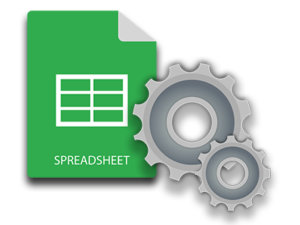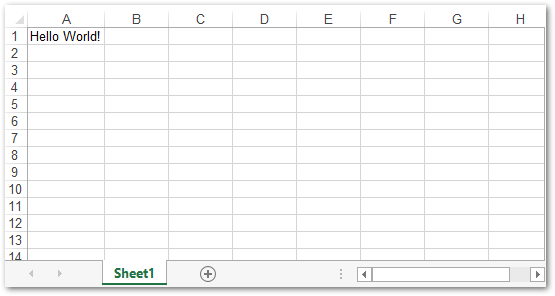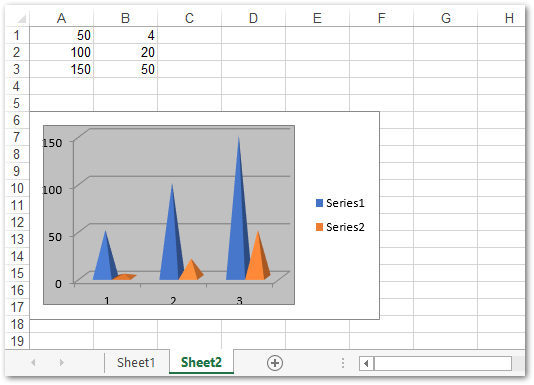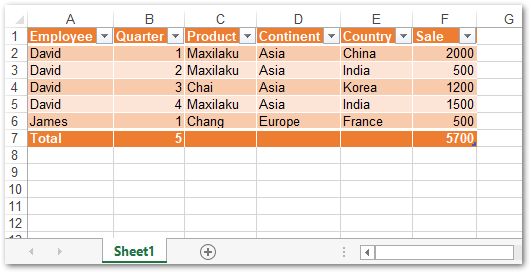## Aspose.Cells使用教程：使用 .NET 在 Linux 上创建或编辑 Excel 文件_aspose.cells文档_Augenstern__zyx的博客-程序员宅基地# 在 Linux 上创建 Excel XLS 或 XLSX

Excel 文件称为工作簿，其中包含一个或多个工作表。这些工作表进一步分为单元格。Aspose.Cells for .NET 使用相同的命名约定来处理 Excel 文件。以下是在 Linux 上从头开始创建新 Excel 文件的步骤。

• 创建Workbook类的实例。
• 使用Workbook.Worksheets集合获取工作簿的第一个工作表（默认创建）的引用。
• 使用单元格的名称从Worksheet.Cells集合中获取所需单元格的引用。
• 添加或更新单元格的值。
• 使用Workbook.Save(string, SaveFormat)方法保存工作簿。

``````// Instantiate a Workbook object that represents Excel file.
Workbook wb = new Workbook();

// When you create a new workbook, a default "Sheet1" is added to the workbook.
Worksheet sheet = wb.Worksheets;

// Access the "A1" cell in the sheet.
Cell cell = sheet.Cells["A1"];

// Input the "Hello World!" text into the "A1" cell.
cell.PutValue("Hello World!");

// Save as .xlsx file.
wb.Save("Excel.xlsx", SaveFormat.Xlsx);
``````# 在 Linux 上将数据写入 Excel XLSX 文件

Aspose.Cells for .NET 还允许您将数据写入现有的 Excel 文件。为此，您可以使用 Excel 文件的路径初始化 Workbook 对象并操作所需的工作表和单元格。以下是在 Linux 上编辑现有 Excel 文件的步骤。

• 创建一个Workbook实例并使用 Excel 文件的路径对其进行初始化。
• 使用Worksheet和Cell类分别访问 Excel 文件中的工作表和单元格。
• 使用Workbook.Save(string, SaveFormat)方法保存工作簿。

``````// Instantiate a Workbook object that represents Excel file.
Workbook wb = new Workbook("Excel.xlsx");

// Access "Sheet1" from the workbook.
Worksheet sheet = wb.Worksheets;

// Access the "A1" cell in the sheet.
Cell cell = sheet.Cells["A1"];

// Input the "Hello World!" text into the "A1" cell.
cell.PutValue("The cell's value is updated.");

// Save the Excel file as .xlsx.
wb.Save("Excel_updated.xlsx", SaveFormat.Xlsx);
``````

# 在 Linux 上的 Excel XLSX 文件中创建图表

• 使用Workbook类创建 Excel 文件。
• 通过访问其单元格将一些数据添加到工作表。
• 通过使用ChartType枚举传递图表类型，使用Worksheet.Charts集合将新图表对象添加到工作表。
• 从Chart对象的集合中获取新创建的图表。
• 指定单元格的范围以向图表对象提供数据源。
• 使用Workbook.Save(string, SaveFormat)方法保存工作簿。

``````// Instantiate a Workbook object that represents Excel file.
Workbook wb = new Workbook();

// Add a new worksheet to the Excel object.

// Obtain the reference of the newly added worksheet by passing its sheet index.
Worksheet worksheet = wb.Worksheets[sheetIndex];

// Add dummy values to cells.
worksheet.Cells["A1"].PutValue(50);
worksheet.Cells["A2"].PutValue(100);
worksheet.Cells["A3"].PutValue(150);
worksheet.Cells["B1"].PutValue(4);
worksheet.Cells["B2"].PutValue(20);
worksheet.Cells["B3"].PutValue(50);

// Add a chart to the worksheet.
int chartIndex = worksheet.Charts.Add(Charts.ChartType.Pyramid, 5, 0, 15, 5);

// Access the instance of the newly added chart.
Charts.Chart chart = worksheet.Charts[chartIndex];

// Add SeriesCollection (chart data source) to the chart ranging from "A1" cell to "B3".

// Save the Excel file.
wb.Save("Excel_Chart.xlsx", SaveFormat.Xlsx);
``````# 在 Linux 上的 Excel XLSX 文件中创建表格

Aspose.Cells for .NET 还提供了一种从工作表中的单元格范围创建表格的简单方法。此外，您可以在表中为总计添加一行。以下是在 Excel 文件中创建表格的步骤。

• 加载 Excel 工作簿或使用Workbook类创建一个新工作簿。
• 将数据添加到工作表的单元格。
• 将新的ListObject添加到工作表。
• 将ListObject.ShowTotals属性设置为true。
• 计算总数并将工作簿保存为 Excel 文件。

``````// Instantiate a Workbook object that represents Excel file.
Workbook wb = new Workbook();

// Get the first worksheet.
Worksheet sheet = wb.Worksheets;

// Obtaining Worksheet's cells collection
Cells cells = sheet.Cells;

// Setting the value to the cells
Aspose.Cells.Cell cell = cells["A1"];
cell.PutValue("Employee");
cell = cells["B1"];
cell.PutValue("Quarter");
cell = cells["C1"];
cell.PutValue("Product");
cell = cells["D1"];
cell.PutValue("Continent");
cell = cells["E1"];
cell.PutValue("Country");
cell = cells["F1"];
cell.PutValue("Sale");

cell = cells["A2"];
cell.PutValue("David");
cell = cells["A3"];
cell.PutValue("David");
cell = cells["A4"];
cell.PutValue("David");
cell = cells["A5"];
cell.PutValue("David");
cell = cells["A6"];
cell.PutValue("James");

cell = cells["B2"];
cell.PutValue(1);
cell = cells["B3"];
cell.PutValue(2);
cell = cells["B4"];
cell.PutValue(3);
cell = cells["B5"];
cell.PutValue(4);
cell = cells["B6"];
cell.PutValue(1);

cell = cells["C2"];
cell.PutValue("Maxilaku");
cell = cells["C3"];
cell.PutValue("Maxilaku");
cell = cells["C4"];
cell.PutValue("Chai");
cell = cells["C5"];
cell.PutValue("Maxilaku");
cell = cells["C6"];
cell.PutValue("Chang");

cell = cells["D2"];
cell.PutValue("Asia");
cell = cells["D3"];
cell.PutValue("Asia");
cell = cells["D4"];
cell.PutValue("Asia");
cell = cells["D5"];
cell.PutValue("Asia");
cell = cells["D6"];
cell.PutValue("Europe");

cell = cells["E2"];
cell.PutValue("China");
cell = cells["E3"];
cell.PutValue("India");
cell = cells["E4"];
cell.PutValue("Korea");
cell = cells["E5"];
cell.PutValue("India");
cell = cells["E6"];
cell.PutValue("France");

cell = cells["F2"];
cell.PutValue(2000);
cell = cells["F3"];
cell.PutValue(500);
cell = cells["F4"];
cell.PutValue(1200);
cell = cells["F5"];
cell.PutValue(1500);
cell = cells["F6"];
cell.PutValue(500);

// Adding a new List Object to the worksheet
Tables.ListObject listObject = sheet.ListObjects[sheet.ListObjects.Add("A1", "F6", true)];

// Adding Default Style to the table
listObject.TableStyleType = Tables.TableStyleType.TableStyleMedium10;

// Show Total
listObject.ShowTotals = true;

// Set the Quarter field's calculation type
listObject.ListColumns.TotalsCalculation = Tables.TotalsCalculation.Count;

// Save the Excel file.
wb.Save("Excel_Table.xlsx", SaveFormat.Xlsx);
``````### Go语言中的一等公民容器类型：Array、Slice和Map-程序员宅基地

Go语言中的一等公民容器类型：Array、Slice和Map在严格意义上，Go中有三种一等公民容器类型：Array、Slice和Map。 有些情况下，String和Channel也被认为是容器，但因其使用方式或原理与另外三种容器存在区别，本文暂不讨论。本文默认读者具有一定的编程基础，因此本文并未详细的梳理全部相关知识点，而是选择了重点或者易错点的内容进行分析。文章目录Go语言中的一等公民容器...

### 2020 ICM Problem E: Drowning in Plastic 翻译_the extent of the current waste problem-程序员宅基地

Since the 1950s, the manufacturing of plastics has grown exponentially because of its variety of uses, such as food packaging, consumer products, medical devices, and construction. While there are sig..._the extent of the current waste problem

### Lesson 2 spare the spider 不要伤害蜘蛛-程序员宅基地

1.New Words各种各样的群flocks and herds 牛群和羊群 flock 多用于羊群 herd 多用于牛群The birds of the same feather flock together. 物以类聚，人以群分You are the same flock. 一丘之貉In the morning , peonle folck into the city.throng an

### hdoj2017 字符串统计 字符串统计--大水题_hdoj字符串匹配-程序员宅基地

#include &lt;iostream&gt;#include &lt;algorithm&gt;#include &lt;map&gt;#include &lt;string&gt; #没有这行会报错using namespace std;int main() { int n; string a; int count; int len;..._hdoj字符串匹配

### hdu1217 floyd算法_纬度打击的博客-程序员宅基地

hdu1217 floyd算法传送门：hdu1217。1.理解题意求有向图有无正环2.思路这一题是是求最短路的变形，且本题的数据并不大，因此可以用floyd算法来求（只需要将加改为乘）。floyd算法的精髓就是dp。3.代码//// Created by wang on 2021/9/16.//hdu1217#include <bits\stdc++.h>using namespace std;typedef long long ll;const int in

### AIX上ulimit -a 输出的含义-程序员宅基地

AIX上ulimit -a 输出的含义 AIX 用户使用的系统资源限制包括两个概念 --- 硬限制(hard limits) 和软限制(soft limits)。 hard limits自AIX 4.1版本开始引入。hard limits 应由AIX系统管理员设置，只有security组的成员可以将此值增大， 用户本身可以减小此限定值,但是其更改将随着该用户从系统退出而失效。使用下列命令可以查看hard limits的限定